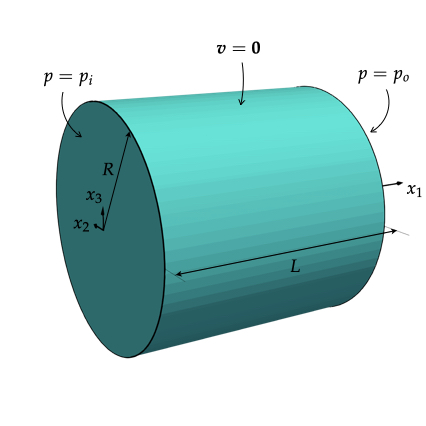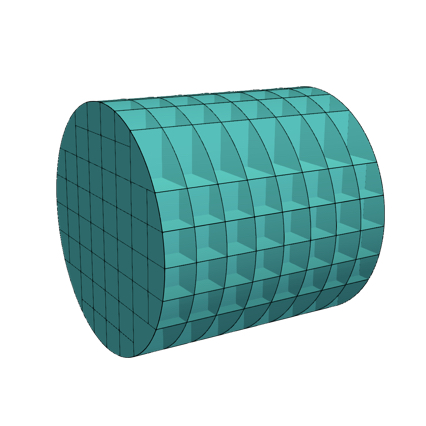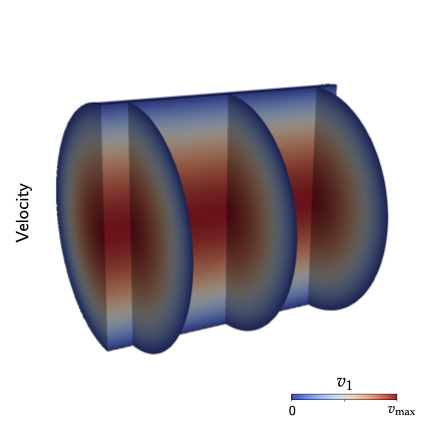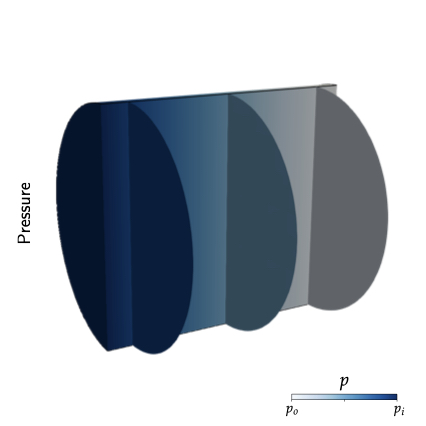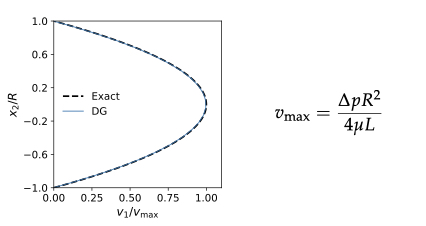Salta al contenuto principale

# hpAMReX

hpAMReX is a collection of software libraries for applications that require hp Adaptive Mesh Refinement algorithms, may run on massively parallel architectures and target eXascale computing. hpAMReX is based on the software framework AMReX

# PySCo

PySCo is a collection of software libraries based on the computer programming language Python for Scientific Computing applications. PySCo features three main modules, namely: Geometry Generation Module, Meshing Tools Module and Solvers Module. Examples on the capabilities of these modules are provided below. In the following sections, all images are generated using ParaView.

### Geometry Generation

PySCo allows the users to generate geometries via explicit parametrizations and/or implicit descriptions (e.g. via level set functions).

#### Example #1: Simple rectangular domain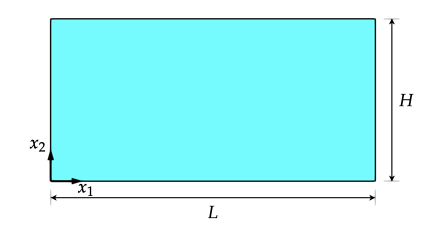Consider a rectangular domain of length L and height H as sketched in the figure above. A generic point x = (x1, x2) in the rectangle may be simply represented as

x = u e1 + v e2

where (u,v) ∈ [0, L] x [0, H].

The code below shows how to introduce such a geometry in PySCo:

 geo = pysco.geometry.base.Geometry(dim = 2) L = 1.0 H = 0.5 geo.add_parametric_surface(ID = "Rectangle", expression = ("u", "v"), domain = ([0.0, L], [0.0, H])

#### Example #2: Cylinder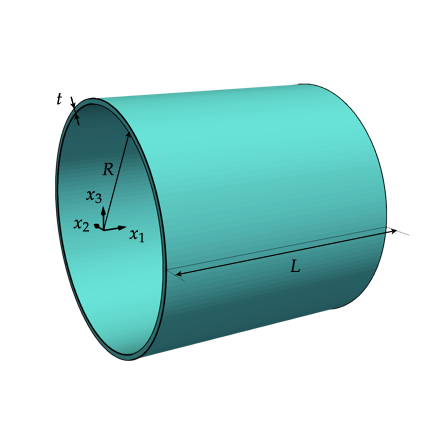A three-dimensional geometry can also be represented in paremetric form. For example, consider a cylinder of length L, radius R and thickness t, as shown above. Its parametric representation may be given as follow

x = u e1 + v cos(w) e2 + v sin(w) e3

where (u,v,w) ∈ [0, L] x [R-t/2, R+t/2] x [0, 2pi].

In PySCo, similar to the preceding example, one uses the following code

 geo = pysco.geometry.base.Geometry(dim = 3) L = 1.0 R = 0.5 t = 0.02 geo.add_parametric_volume(ID = "Cylinder", expression = ("u", "v*cos(w), "v*sin(w)"), domain = ([0.0, L], [R-0.5*t, R+0.5*t], [0.0, 2*pi])

#### Example #3: NURBS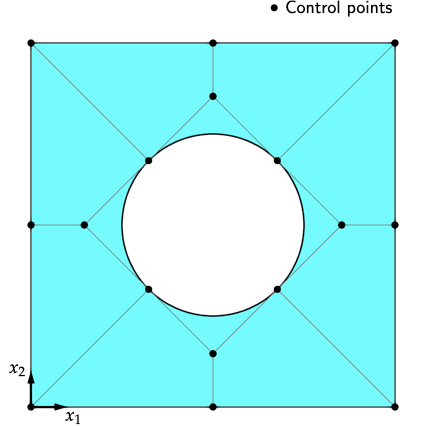### Meshing Tools

PySCo supports (adaptive) structured grids with embedded boundaries, polygonal and polyhedral meshes and triangular and tetrahedral meshes. PySCo also provides an interface with the meshing software library Gmsh.

#### Example #1: Structured mesh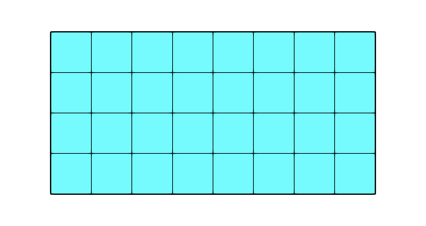Parametric surfaces and volumes can be easily partitioned using structured grids. The figure above shows the 8 x 4 structured mesh of the rectangular domain of the Example #1 in the Geometry Generation section. In Pysco, such a domain partition is performed through the following code

 geo.add_parametric_surface(ID = "Rectangle", expression = ("u", "v"), domain = ([0.0, L], [0.0, H]) geo.init_surface_mesh()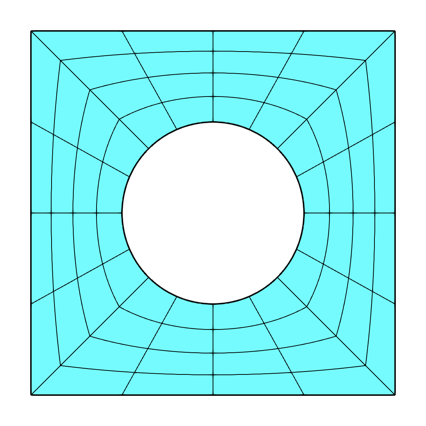#### Example #2: Embedded-boundary mesh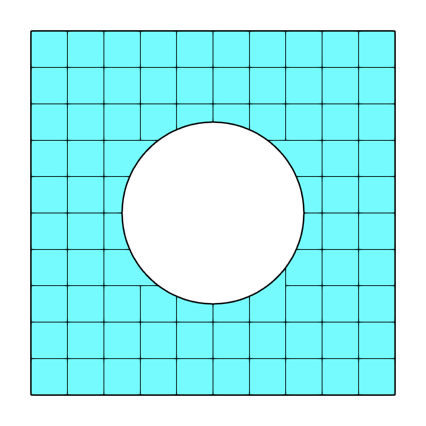#### Example #3: Polygonal mesh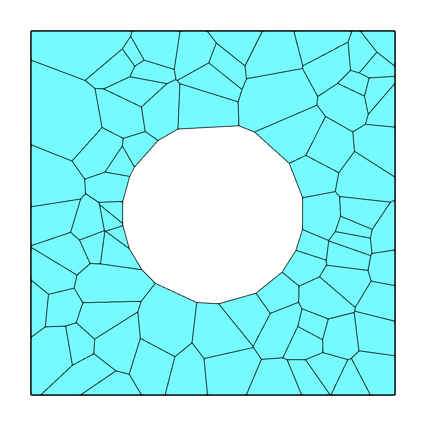Neper

### Solvers

PySCo allows solving Partial Differential Equations (PDEs) using Discontinuous Galerkin (DG) methods.

#### Example #1: Stokes flow 2D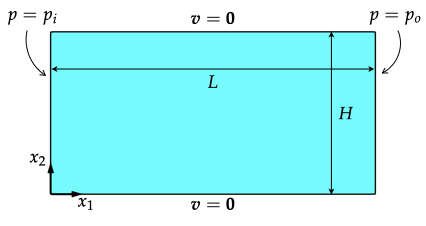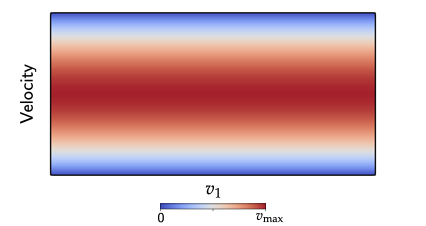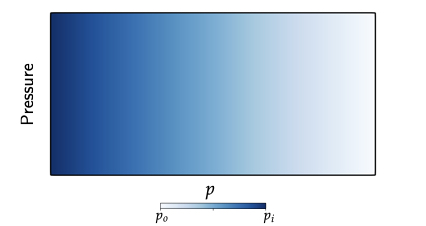#### Example #2: Stokes flow 3D